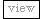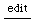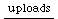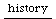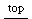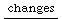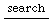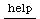## McCartney-Voss-Algorithm for 1/f Noise

These algorithms use a binary number representation to decide which generator is updated.
This is emulated here, which is inefficient, but demonstrates the way the
Duty UGen works. Duty takes the duration as first argument, which is calculated in seconds - SampleDur is a ugen that returns the number of seconds a sample takes (like e.g. 1/48000)

```

// Voss-Algorithm

xxxxxxxxxxxxxxxx
x x x x x x x xx
x   x   x   x  x
x       x      x

i % 0
i % 1
i % 2
i % 4

// pink noise: explicitly generated by demand ugen
(
{
var dt, n, g;
dt = SampleDur.ir;
n = 4;

g = {|i|
Duty.ar(
(2 ** i * dt),
0,
Dwhite(-1, 1, inf)
)
} ! n;
g.sum * 0.1 / n

}.play
)
// built in
{ PinkNoise.ar * 0.1 }.play;

```

```// Voss-McCartney-Algorithm (9/1999)

xxxxxxxxxxxxxxxxxxxx
x x x x x x x xx xx
x   x   x   x  x
x       x      x

i % 0 + 0
i % 1 + 1
i % 2 + 2
i % 4 + 4

(
{
var dt, n, g;
dt = SampleDur.ir;
n = 4;

g = {|i|
var z;
z = 2 ** i * dt;
Duty.ar(
z * Dseq([2, Dseries(1,0,inf)]), // offset. this returns the values: z * 2,1,1,1,1,1,1,...
0,
Dwhite(-1, 1, inf)
)
} ! n;
g.sum * 0.1 / n

}.play
)

{ PinkNoise.ar * 0.1 }.play;

// random offests:

{
var dt, n, g;
dt = SampleDur.ir;
n = 4;

g = {|i|
var z;
z = 2 ** i * dt;
Duty.ar(
Dwhite(0, z, inf), // random offset.
0,
Dwhite(-1, 1, inf)
)
} ! n;
g.sum * 0.1 / n

}.play

```

there is some more discussion and a further refinement of this algorithm which can be found here:

jrh